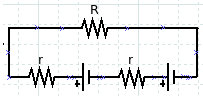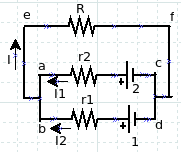# EMFs in series and parallelEMFs in series and parallel

If there are two or more sources of electromotive (emf) connected as shown in the figure, the emf is arranged in series.

The equivalent voltage source (ε) is:

ε = ε1 + ε2 + εn

The equivalent internal resistance (r) is:

r = r1 + r2 + rn

The electric current flowing through the external resistance (R) is:

I = ε / (r + R)

Sample problem:

Suppose that two batteries each emf is 1.5 Volt and the internal resistance value in each battery is 0.1 Ω. External resistance (R) = 10 Ω. The direction of the electric current clockwise.

Use the previous formula:

ε = 1.5 + 1.5 = 3 Volt

r = 0.1 + 0.1 = 0.2 Ω

I = ε / (r + R) = 3 / (0.2 + 10)

I = 3 / 10.2

I = 0.294 A

Use Kirchhoff’s second rule:

1.5 – 0.1 I + 1.5 – 0.1 I – 10 I = 0

3 – 0.2 I – 10 I = 0

3 – 10.2 I = 0

3 = 10.2 I

I = 3 / 10.2

I = 0.294 A

If there are two or more sources of electromotive (emf) connected as shown in the figure, the emf is connected in parallel.

The equivalent voltage source (ε) is:ε = ε1 = ε2 = εn

The equivalent internal resistance (r) is:

1/r = 1/r1 + 1/r2 + 1/rn

The electric current flowing through the external resistance (R) is:

I = ε / (r + R)

Sample problem:

Suppose that two batteries each emf is 1.5 Volt and the resistance value in each battery is 0.1 Ω. External resistance (R) = 10 Ω.

Use the previous formula:

ε = 1.5 Volt

1/r = 1/0.1 + 1/0.1 = 2 / 0.1

r = 0.1 / 2 = 0.05 Ω

I = ε / (r + R) = 1.5 / (0.05 + 10) = 1.5 / 10.05

I = 0.149 A

Use Kirchhoff’s rule

Apply Kirchhoff‘s first rule:

I1 + I2 = I ………. Equation 1

Analyze Aefca loop. The direction of the loop is clockwise. Apply Kirchhoff’s second rule:

ε2 – I1 r2 – I R = 0

1.5 – 0.1 I1 – 10 I = 0

– 0.1 I1 = 10 I – 1.5

I1 = (10 I – 1.5) / – 0.1

I1 = -100 I + 15 ………. Equation 2

Analyze the Befdb loop. The direction of the loop is clockwise. Apply Kirchhoff’s second law:

ε1 – I2 r1 – I R = 0

1.5 – 0.1 I2 – 10 I = 0

0.1 I2 = 10 I – 1.5

I2 = (10 I – 1.5) / – 0.1

I2 = -100 I + 15 ………. Equation 3

Substitute equation 2 and 3 to equation 1:

I1 + I2 = I

-100 I + 15 – 100 I + 15 = I

– 200 I + 30 = I

30 = I + 200 I

30 = 201 I

I = 30 / 201

I = 0.149 A

Eliminate equation 2 and 3:

I1 = -100 I + 15

I2 = -100 I + 15

——————– –

I1 – I2 = 0

I1 = I2 ………. Equation 4

Because I1 + I2 = I, where I1 = I2 then I1 = I2 = 1/2 I = 1/2 (0.149) = 0.0745 A.